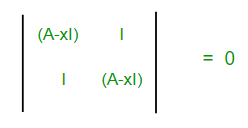Related Articles
GATE | GATE-CS-2007 | Question 25
• Difficulty Level : Expert
• Last Updated : 14 Feb, 2018

Let A be a 4 x 4 matrix with eigenvalues -5, -2, 1, 4. Which of the following is an eigenvalue of

```[A  I]
[I  A] ```

where I is the 4 x 4 identity matrix?
(A) -5
(B) -7
(C) 2
(D) 1

Explanation:
We find the eigenvalues of given matrix by solving its characteristic equation :|(A – x I)2 – I2| = 0
|(A – (x – 1) I) * (A – (x + 1) I)| = 0
|(A – (x – 1) I)| * |(A – (x + 1) I)| = 0
So, |(A – (x – 1) I)| = 0 or |(A – (x + 1) I)| = 0

Let y be eigenvalues of A, then |(A – y I)| = 0 .

So, by comparing equations we get,
either x – 1 = y or x + 1 = y

Therefore , x = y + 1 or x = y – 1

y = -5, -4, 1, 4 (given)

So, x = −4, −1, 2, 5, −6, −3, 0, 3

Thus, option (C) is the answer.

Please comment below if you find anything wrong in the above post.

Quiz of this QuestionMy Personal Notes arrow_drop_up
Recommended Articles
Page :